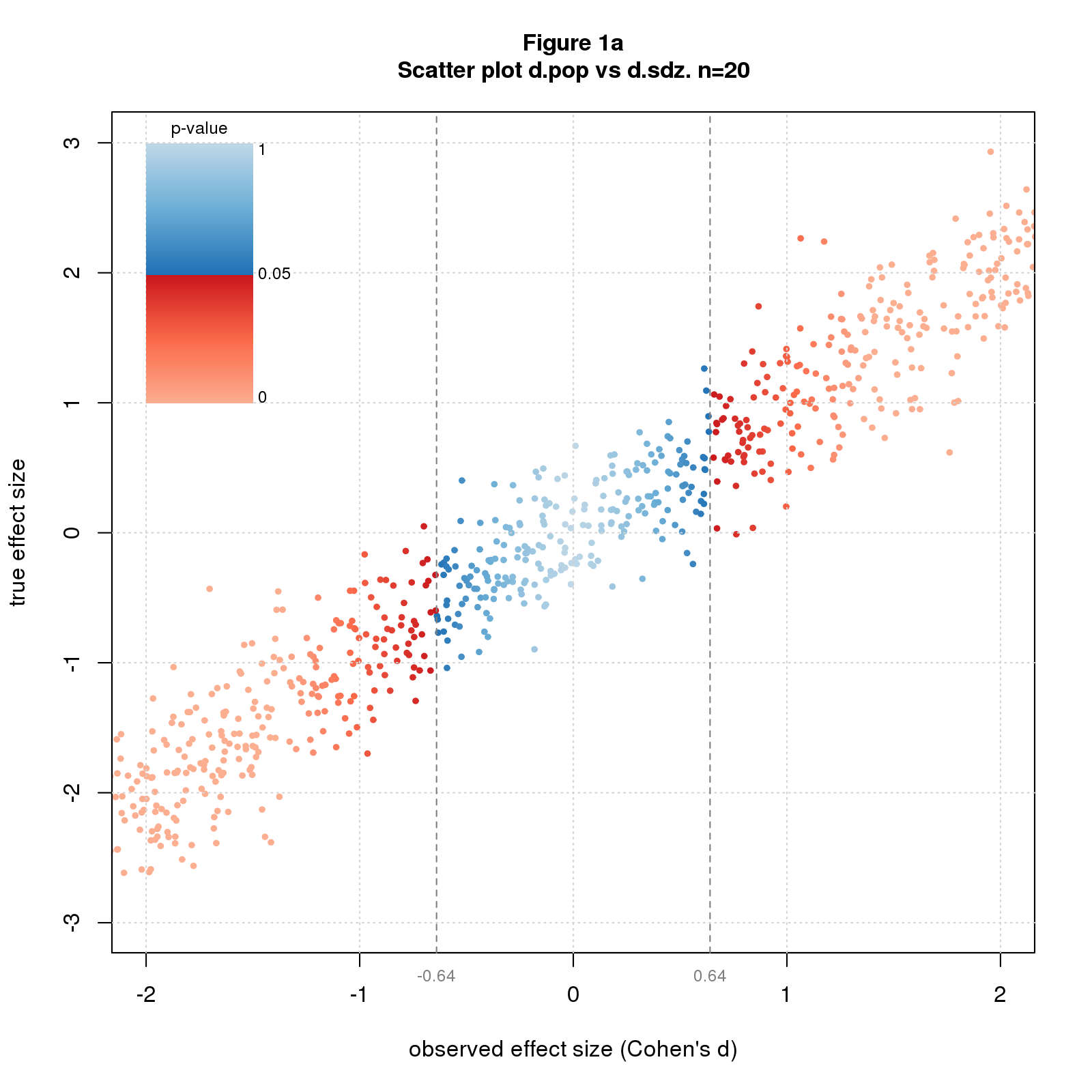A collection of R scripts and documents exploring mistakes made by significance testing. The content at present are

THE SOFTWARE IS A WORK IN PROGRESS. THE CODE IS ROUGH and SOFTWARE DOCUMENTATION NONEXISTENT. PLEASE GET IN TOUCH IF YOU NEED HELP

## Installation and Usage

The software is not a package and cannot be installed by `devtools::install_github` or related. Sorry. The simplest way to get the software is to download or clone the entire repo.

The code mostly uses base R capabilities but has a few dependencies: `RColorBrewer`, `akima`, `knitr`, and `pryr`. Since it’s not a package, you have to manually install these packages if you don’t already have them.

The recommended way to run the program is to `source` the file `R/run.R` into your R session; `R/run.R` will source the rest. Once loaded, you can run the program by executing the statement `run()` as shown below.

``````## This code block assumes your working directory is the root of the distribution

source('R/run.R');
run();``````

This runs the program in a demo-like mode that quickly generates the data and produces the figures and table that appear in this README document. The default computation runs three kinds of simulations totaling 47,000 instances, generates 10 other small data files, and produces 8 figures and one table. The data generation takes about 11 seconds on my small Linux server; the figures and table take about 20 seconds, much of which is spent rendering the plots over a remote X11 connection.

The code that generates the data is in `R/dat_readme.R`; the code that creates the figures and table is in `R/doc_readme.R`.

You can run each part separately by running one or more of the statements below.

``````## This code block assumes your working directory is the root of the distribution

init(doc='readme');          # you MUST specify doc to run the program in pieces
dodat();                     # generate data
dodoc();                     # generate figures and tables
dodoc(save.out=F);           # generate figures and tables without saving them
dodoc(figscreen=F);          # generate and save figures without plotting to screen. much faster!``````

The program can also generate the data and outputs for the documents associated with the project. To generate these, execute `run()` with a suitable `doc` argument as shown below.

``````## This code block assumes your working directory is the root of the distribution.

source('R/run.R');
run(doc='ovrfx');            # run code for blog post on significant effect size inflation``````

The `doc` arguments for each document are

document `doc` argument
When You Select Significant Findings, You’re Selecting Inflated Estimates `ovrfx`
Your P-Values are Too Small! And So Are Your Confidence Intervals! `ovrht`
README `readme`
Supplementary Material for README `readmesupp`
supplementary material for blog posts TBD TBD

## Nomenclature

in code in text meaning
`n` \(n\) sample size
`d.pop` \(d_{pop}\) population effect size
`d.sdz` \(d_{sdz}\) standardized observed effect size, aka Cohen’s d
`d.het` \(d_{het}\) mean of heterogeneous population distribution
`sd.het` \(sd_{het}\) standard deviation of heterogeneous population distribution
`d` \(d\) variously means either \(d_{pop}\), \(d_{sdz}\), or \(d_{het}\); hopefully clear from context
`meand.simu` \(meand_{simu}\) mean significant observed effect size computed from simulated data
`meand.theo` \(meand_{theo}\) mean significant observed effect size computed analytically
`pval.simu` \(pval_{simu}\) p-value computed from simulated data
`pval.theo` \(pval_{theo}\) p-value computed analytically

The program performs three types of simulations.

1. fixd. Fixes \(d_{pop}\) to a few values of interest, sets \(n\) to a range of values, and simulates many studies for each \(d_{pop}\) and \(n\).
2. rand. Randomly selects a large number of \(d_{pop}\)s, sets \(n\) to a range of values, and simulates one study for each \(d_{pop}\) and \(n\).
3. hetd. Sets \(d_{het}\) and \(sd_{het}\) to a few values of interest, and selects a large number of \(d_{pop}\)s from normal distributions with mean \(d_{het}\) and standard deviation \(sd_{het}\). Then sets \(n\) to a range of values, and simulates one study for each \(d_{pop}\) and \(n\).

The program implements two data generation models:

1. fixed effect (fix) which imagines we’re repeating a study an infinite number of times, drawing a different sample each time from a population with a fixed true effect size
2. heterogeneous effect (het) which assumes that each time we do the study, we’re sampling from a different population with a different true effect size

## Figures and Tables

The default mode produces figures that illustrate the kinds of graphs the program can produce.

1. \(d\) vs. \(d\) scatter plot colored by p-value; by default \(d_{pop}\) vs. \(d_{sdz}\)
2. histogram typically of \(d_{sdz}\) colored by p-value
3. probability distribution vs. \(d_{sdz}\) colored by p-value; can compute probability density or cumulative probability internally, or you can pass the distribution into the function
4. multiple line plot; my adaptation of R’s `matplot`

The first figure shows a \(d\) vs. \(d\) scatter plot of rand simulated data for \(n=20\). The second uses the same data but zooms in to the critical region where p-values switch from nonsignificant to significant.